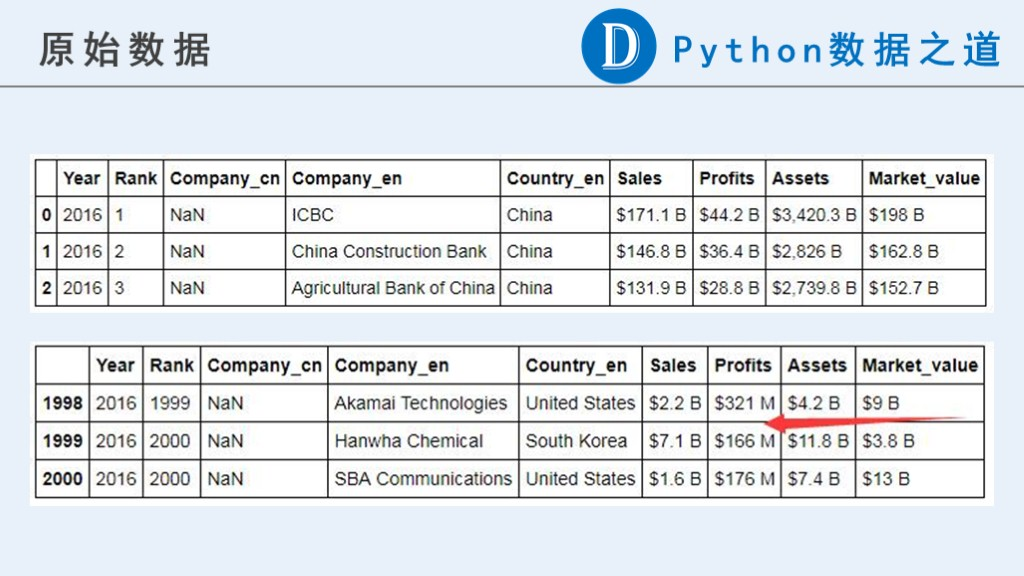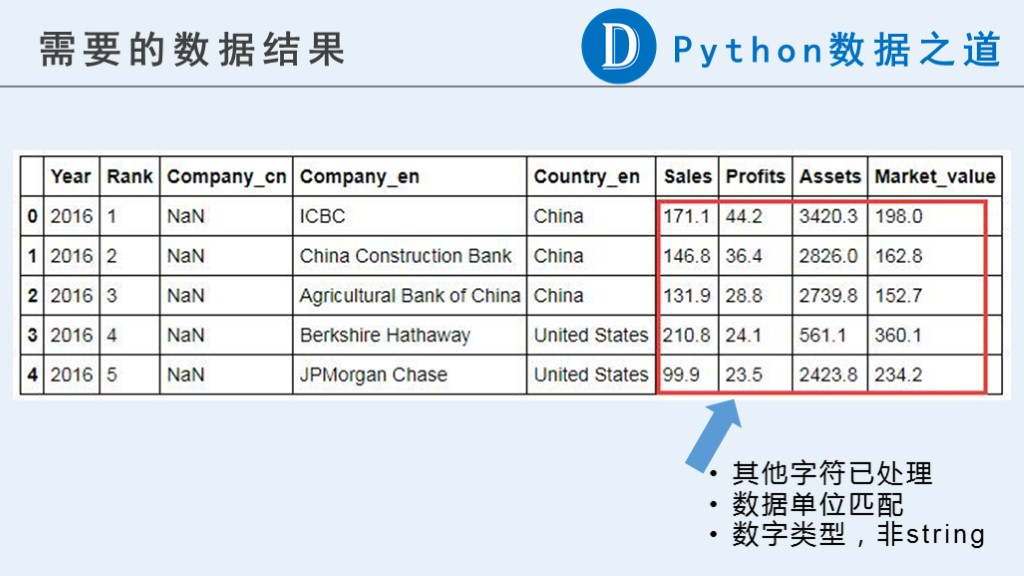# 福布斯系列(8)-数据清洗(d)

projects-forbes Pandas Numpy

Pandas数据处理实战：福布斯全球上市企业排行榜数据整理

• windows 7， 64位
• python 3.5
• pandas 0.19.2版本### 2.3 处理’M’相关的数据

（2）替换字符串“M”为空值

（3）用pd.to_numeric()转换为数字

（4）除以1000，转换为十亿美元，与其他行的数据一致

# 替换美元符号
df_2016['Sales'] = df_2016['Sales'].str.replace('$','') # # 查看异常值，均为字母（“undefined”） # df_2016[df_2016['Sales'].str.isalpha()] # 替换异常值“undefined”为空白 # df_2016['Sales'] = df_2016['Sales'].str.replace('undefined','') df_2016['Sales'] = df_2016['Sales'].str.replace('^[A-Za-z]+$','')

# 替换符号十亿美元“B”为空白，数字本身代表的就是十亿美元为单位
df_2016['Sales'] = df_2016['Sales'].str.replace('B','')

# 处理含有百万“M”为单位的数据，即以“M”结尾的数据
# 思路：
# （2）替换字符串“M”为空值
# （3）用pd.to_numeric()转换为数字
# （4）除以1000，转换为十亿美元，与其他行的数据一致

df_2016['Sales'] = pd.to_numeric(df_2016['Sales'])
print('the shape of DataFrame: ', df_2016.shape)
print(df_2016.dtypes)


## 3 处理方法 Method-3

### 3.2 编写数据处理的自定义函数

def pro_col(df, col):
# 替换相关字符串，如有更多的替换情形，可以自行添加
df[col] = df[col].str.replace('$','') df[col] = df[col].str.replace('^[A-Za-z]+$','')
df[col] = df[col].str.replace('B','')

# 注意这里是'-$'，即以'-'结尾，而不是'-'，因为有负数 df[col] = df[col].str.replace('-$','')
df[col] = df[col].str.replace(',','')

# 处理含有百万“M”为单位的数据，即以“M”结尾的数据
# 思路：
# （2）替换字符串“M”为空值
# （3）用pd.to_numeric()转换为数字
# （4）除以1000，转换为十亿美元，与其他行的数据一致

# 将字符型的数字转换为数字类型
df[col] = pd.to_numeric(df[col])
return df


### 3.3 将自定义函数进行应用

pro_col(df_2016, 'Sales')
pro_col(df_2016, 'Profits')
pro_col(df_2016, 'Assets')
pro_col(df_2016, 'Market_value')

print('the shape of DataFrame: ', df_2016.shape)
print(df_2016.dtypes)


cols = ['Sales', 'Profits', 'Assets', 'Market_value']
for col in cols:
pro_col(df_2016, col)

print('the shape of DataFrame: ', df_2016.shape)
print(df_2016.dtypes)## 本文历史

• 2017-09-05，修改文章标题
• 2017-07-10，本文首发于微信公众号